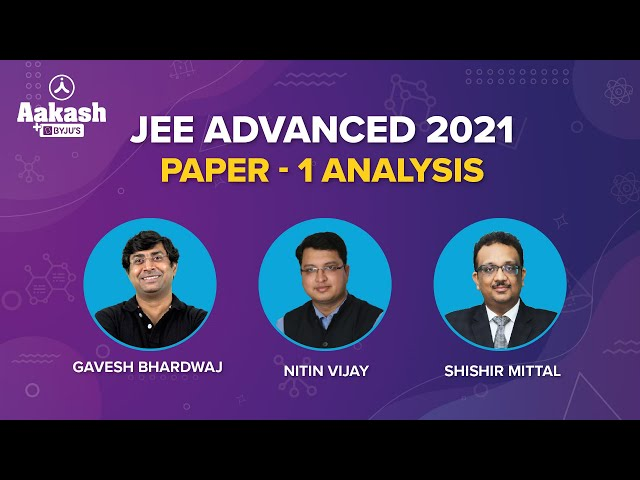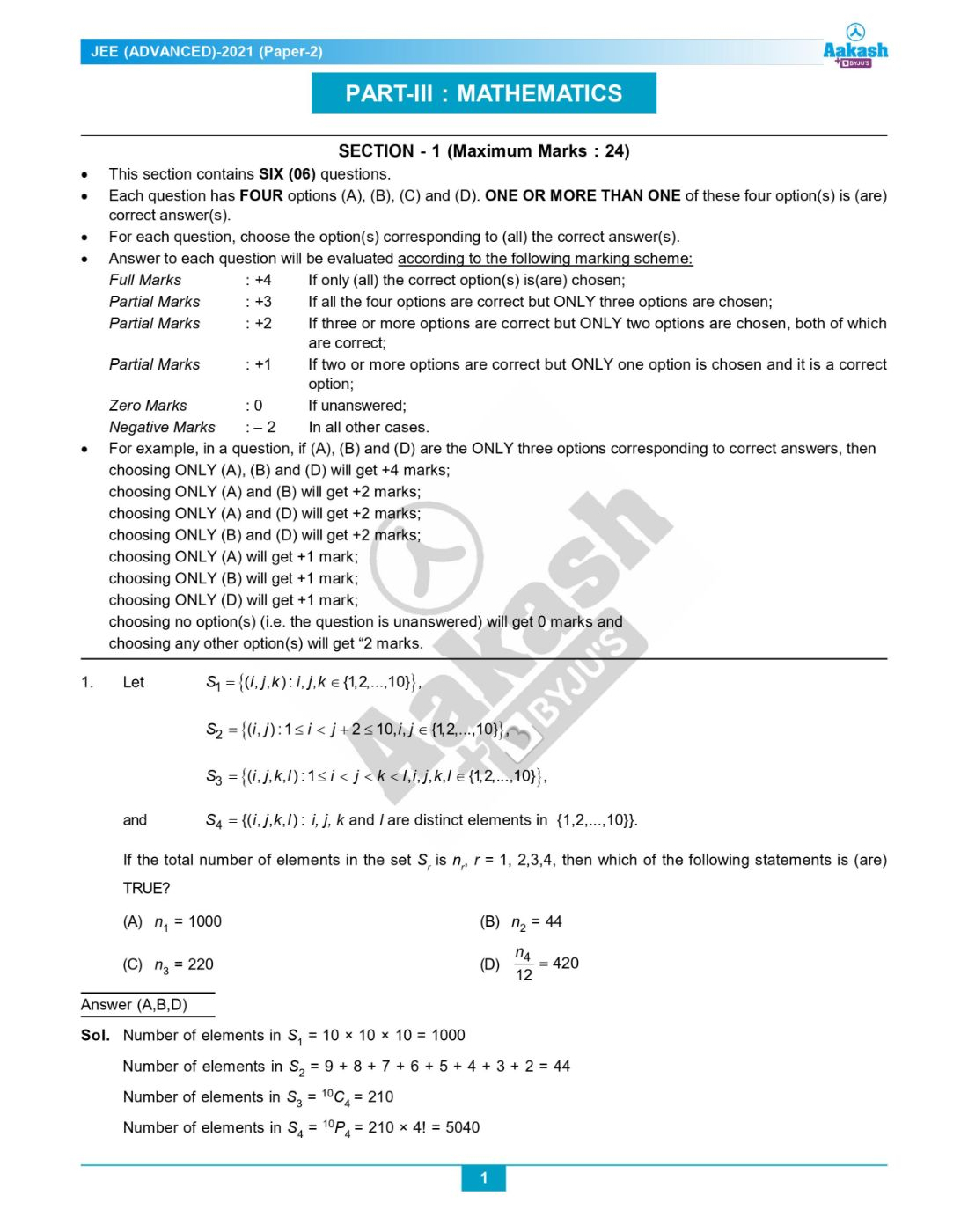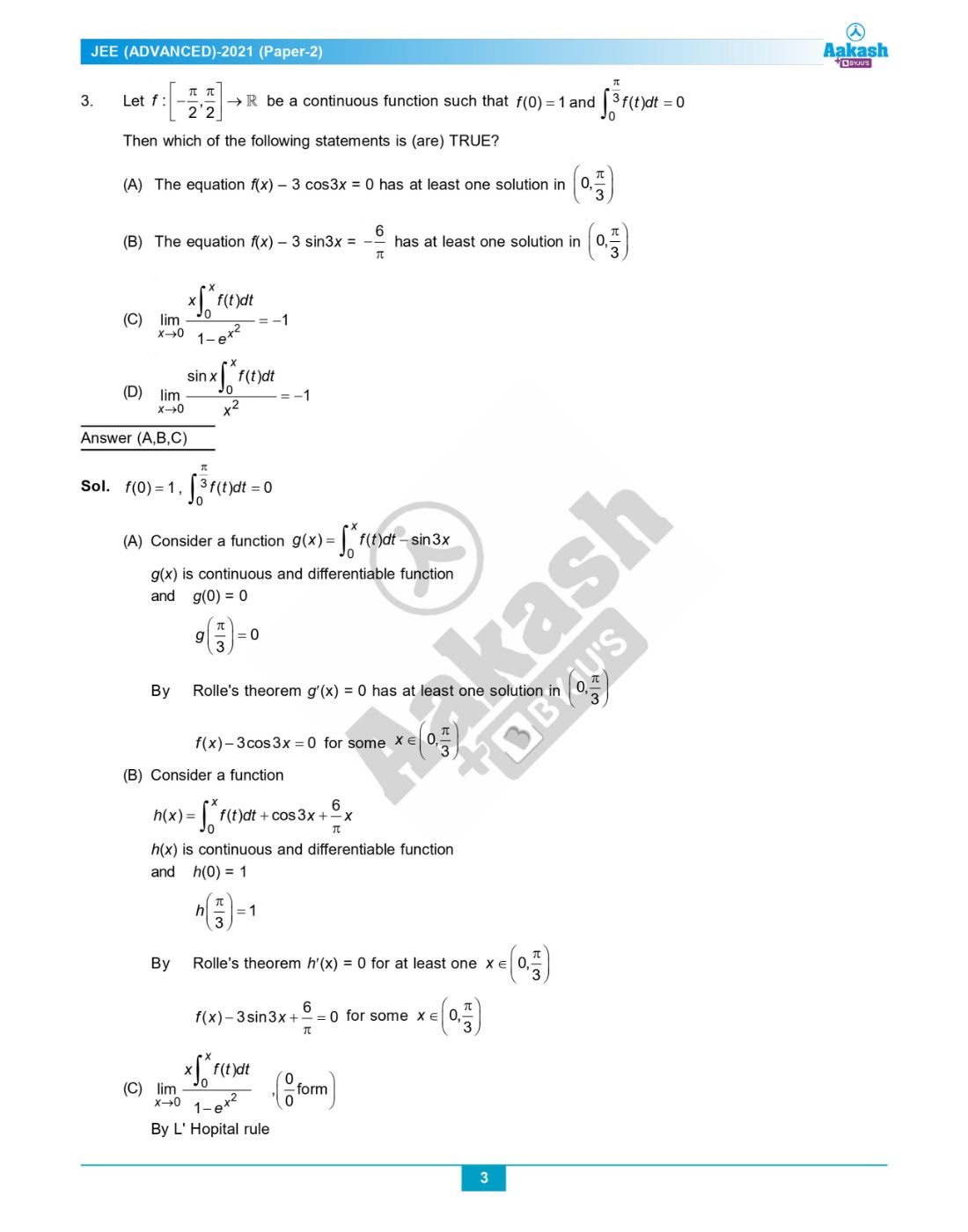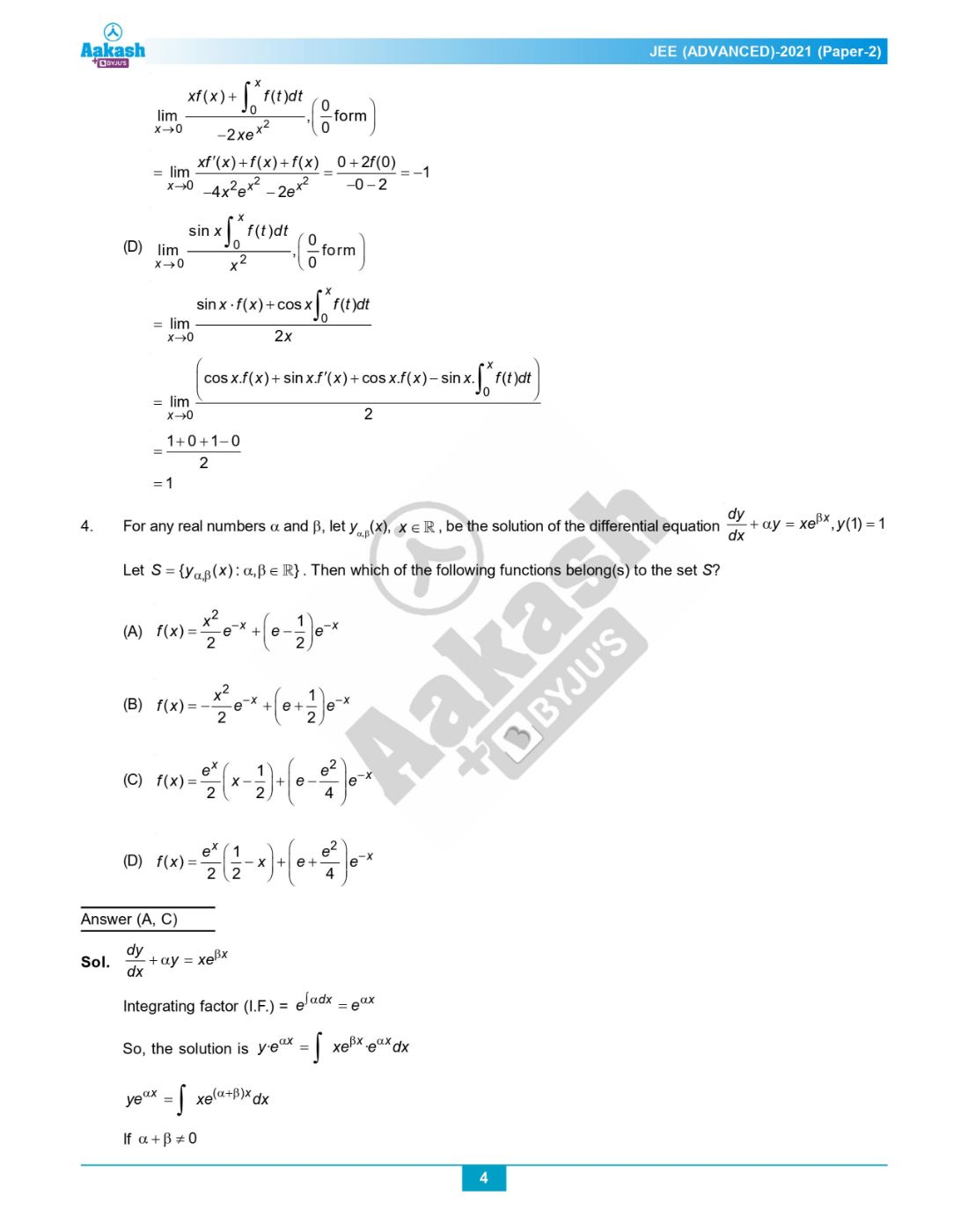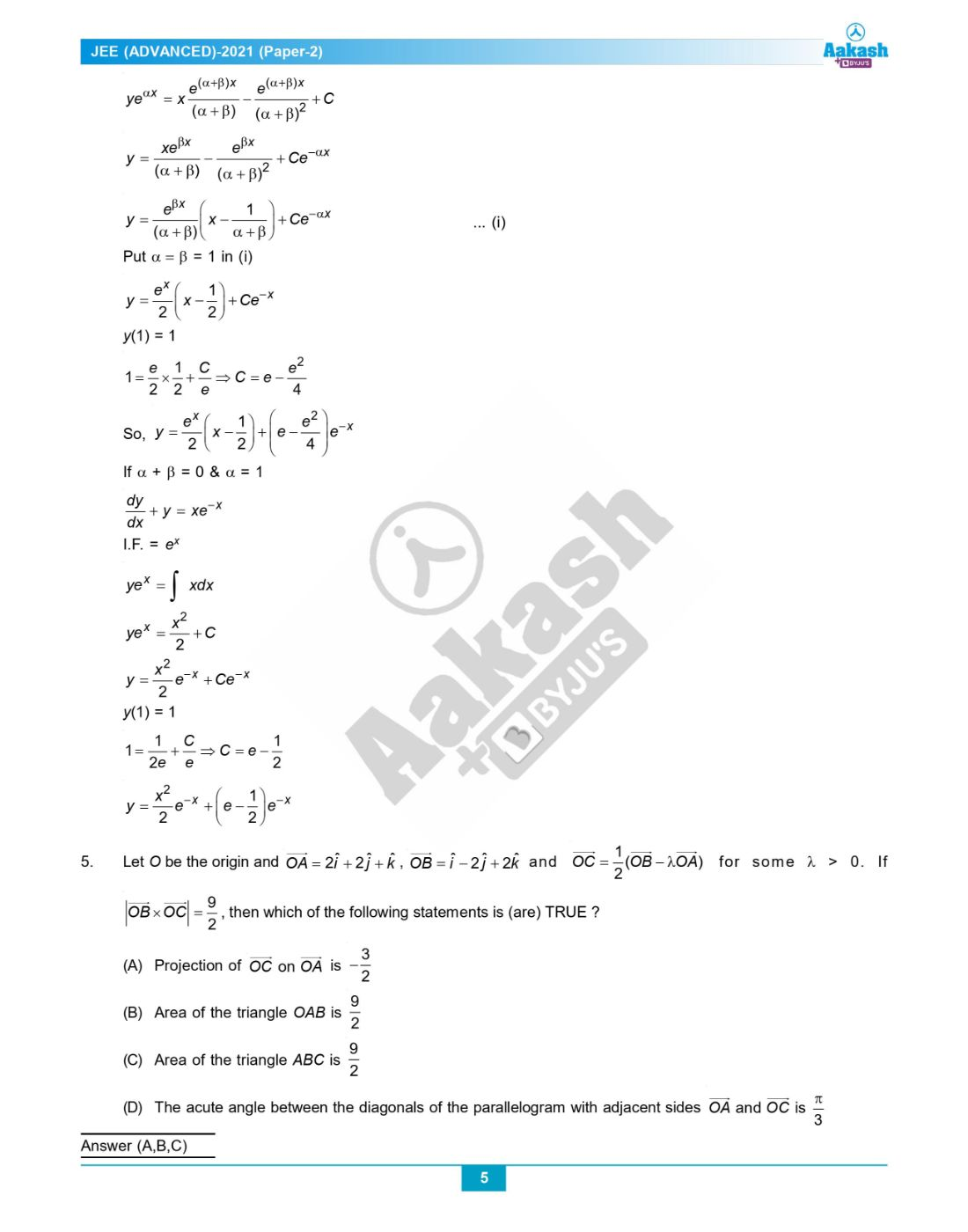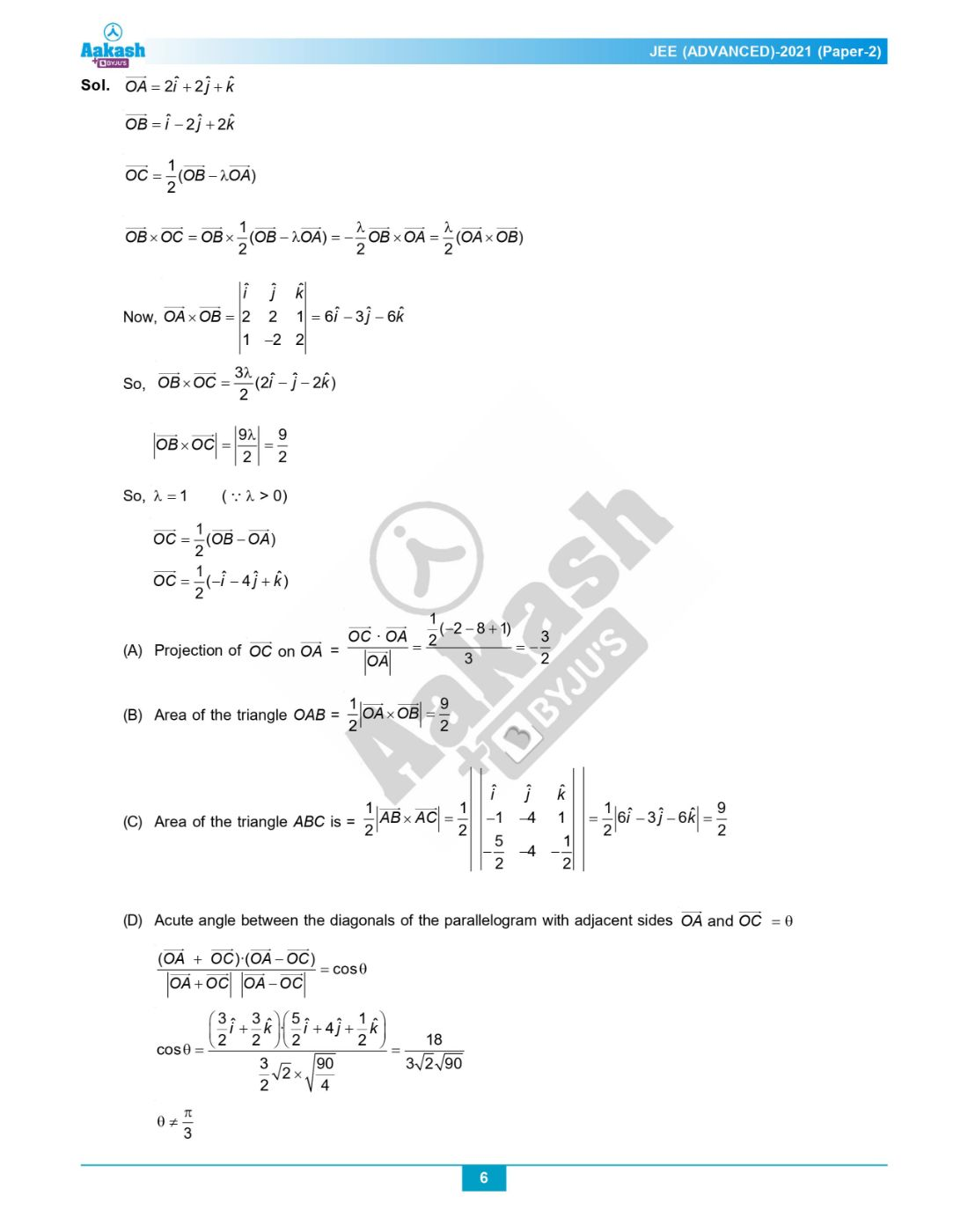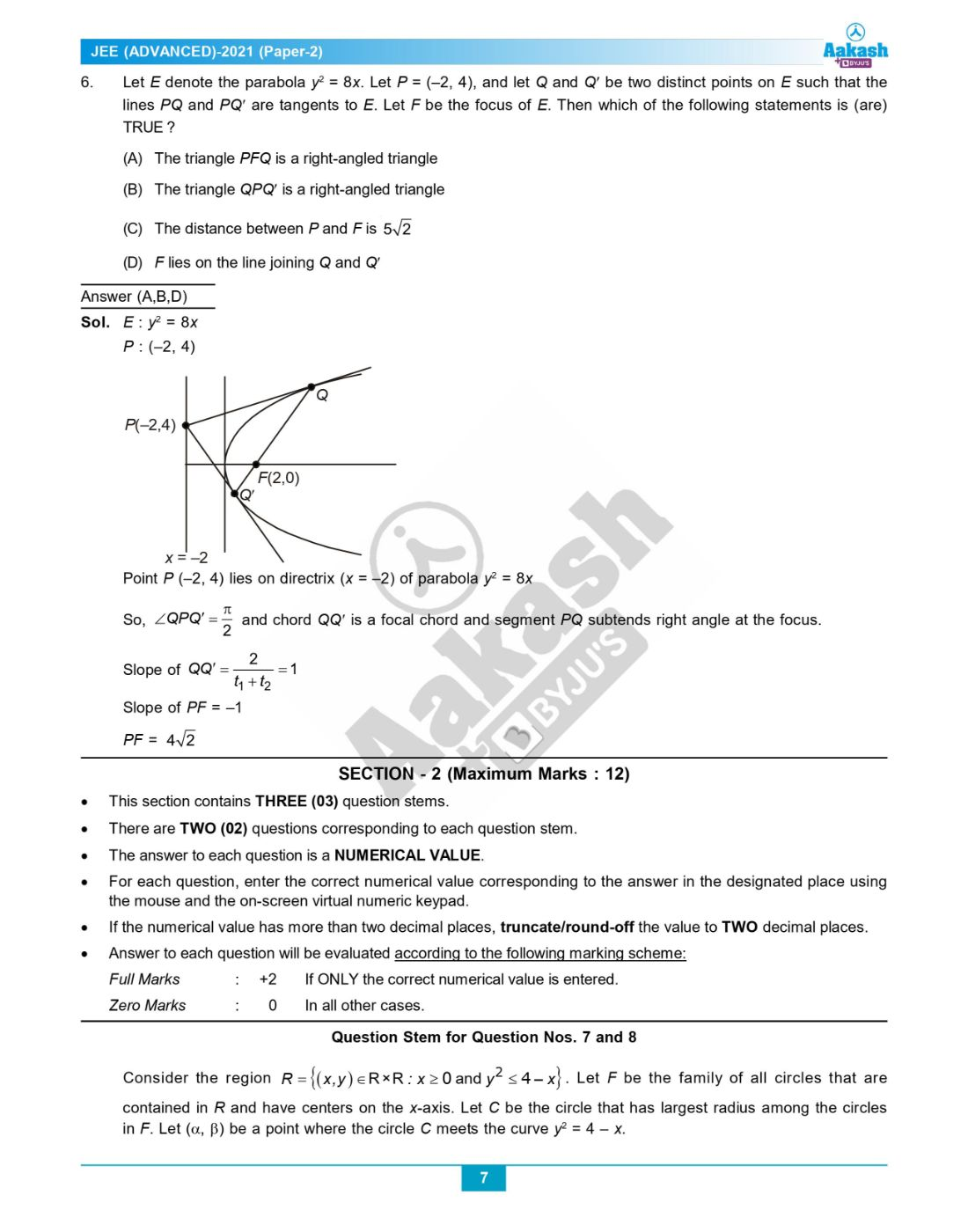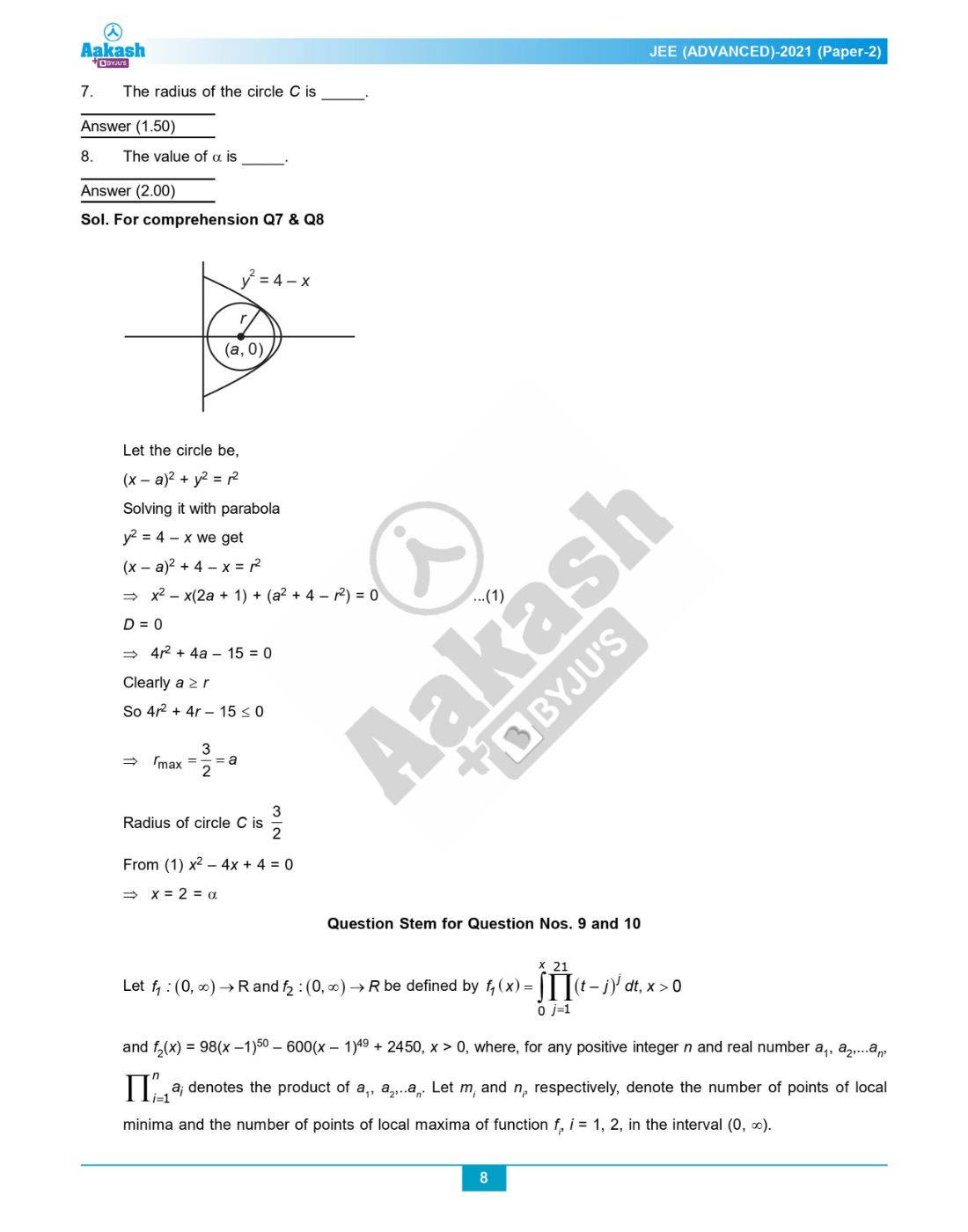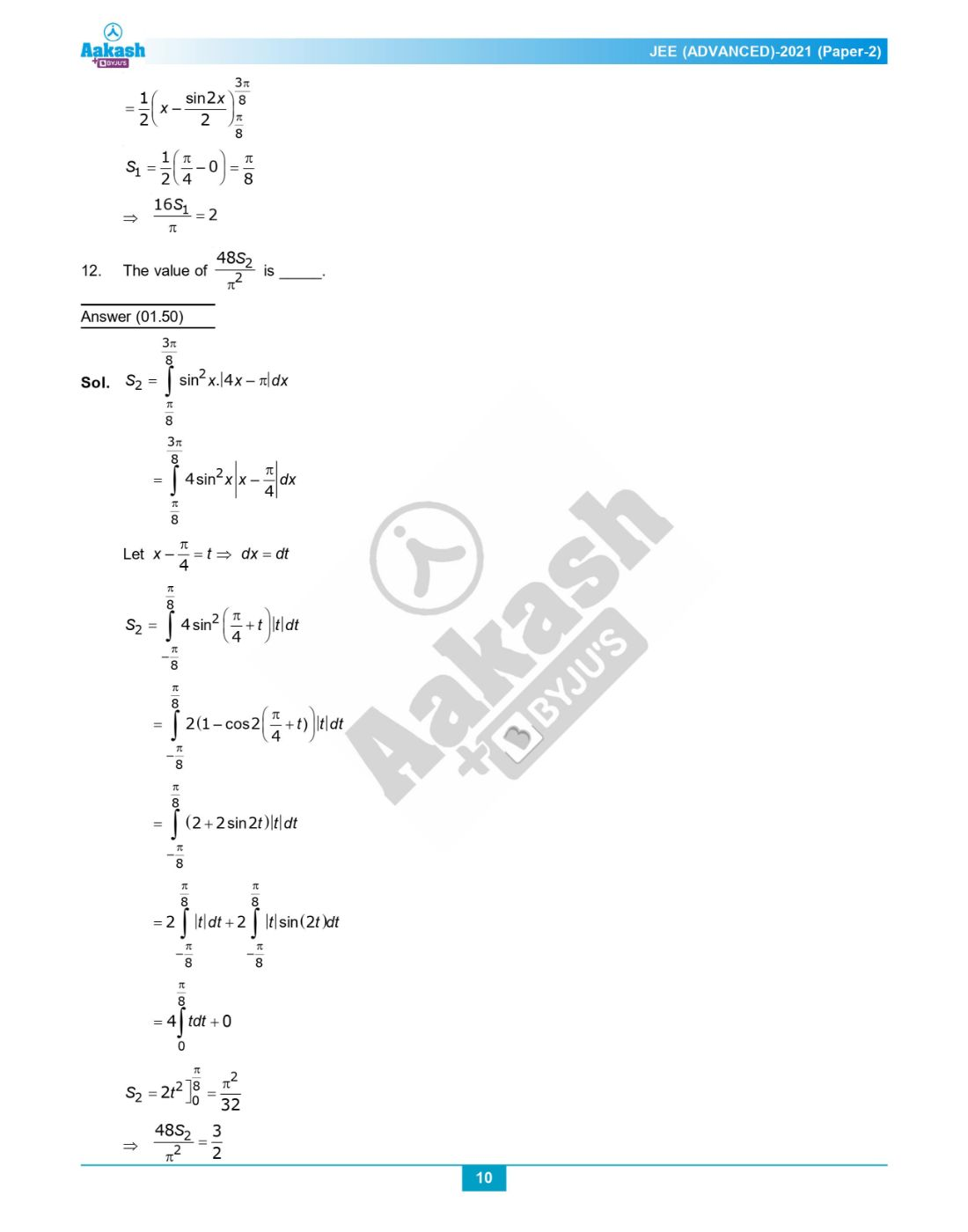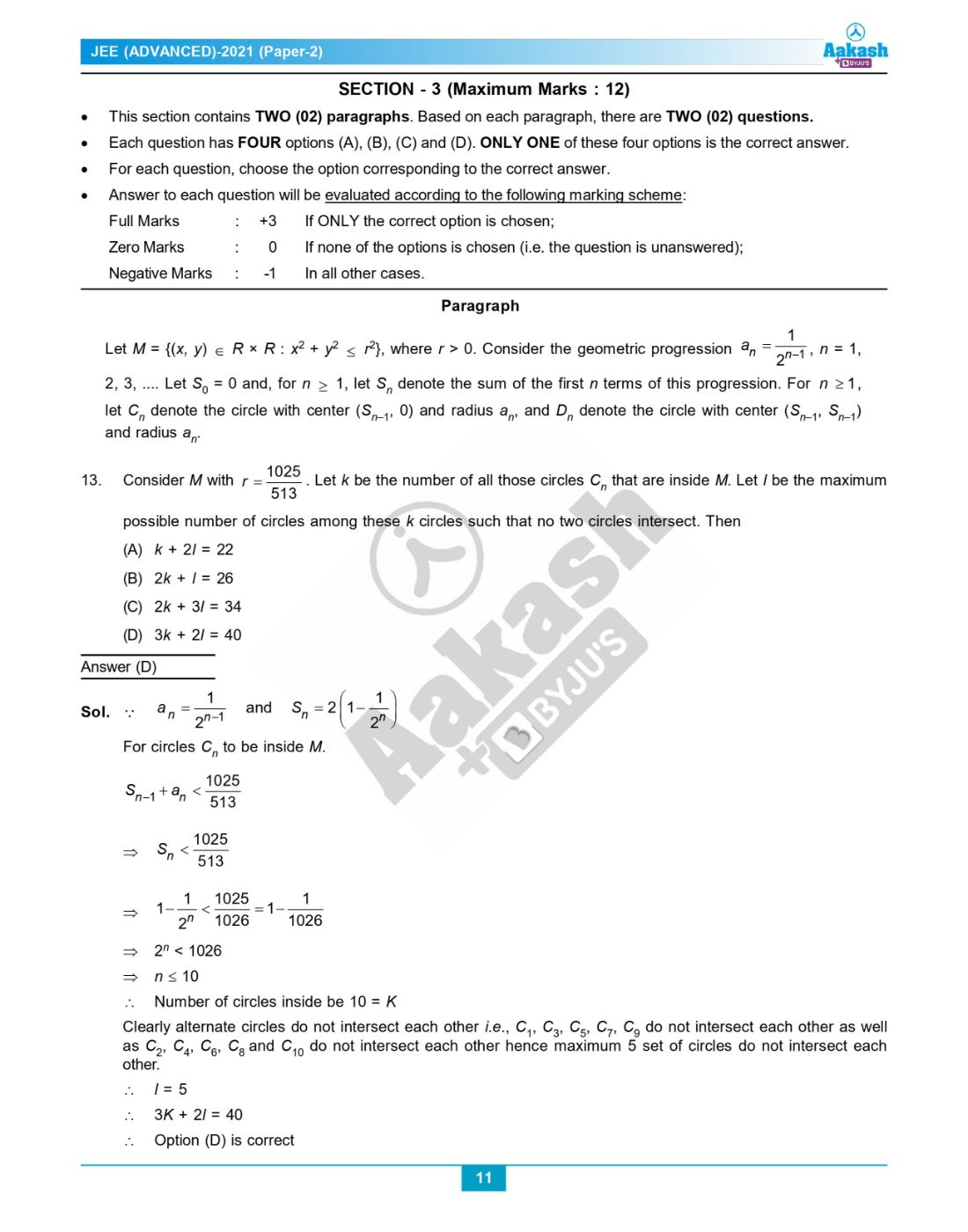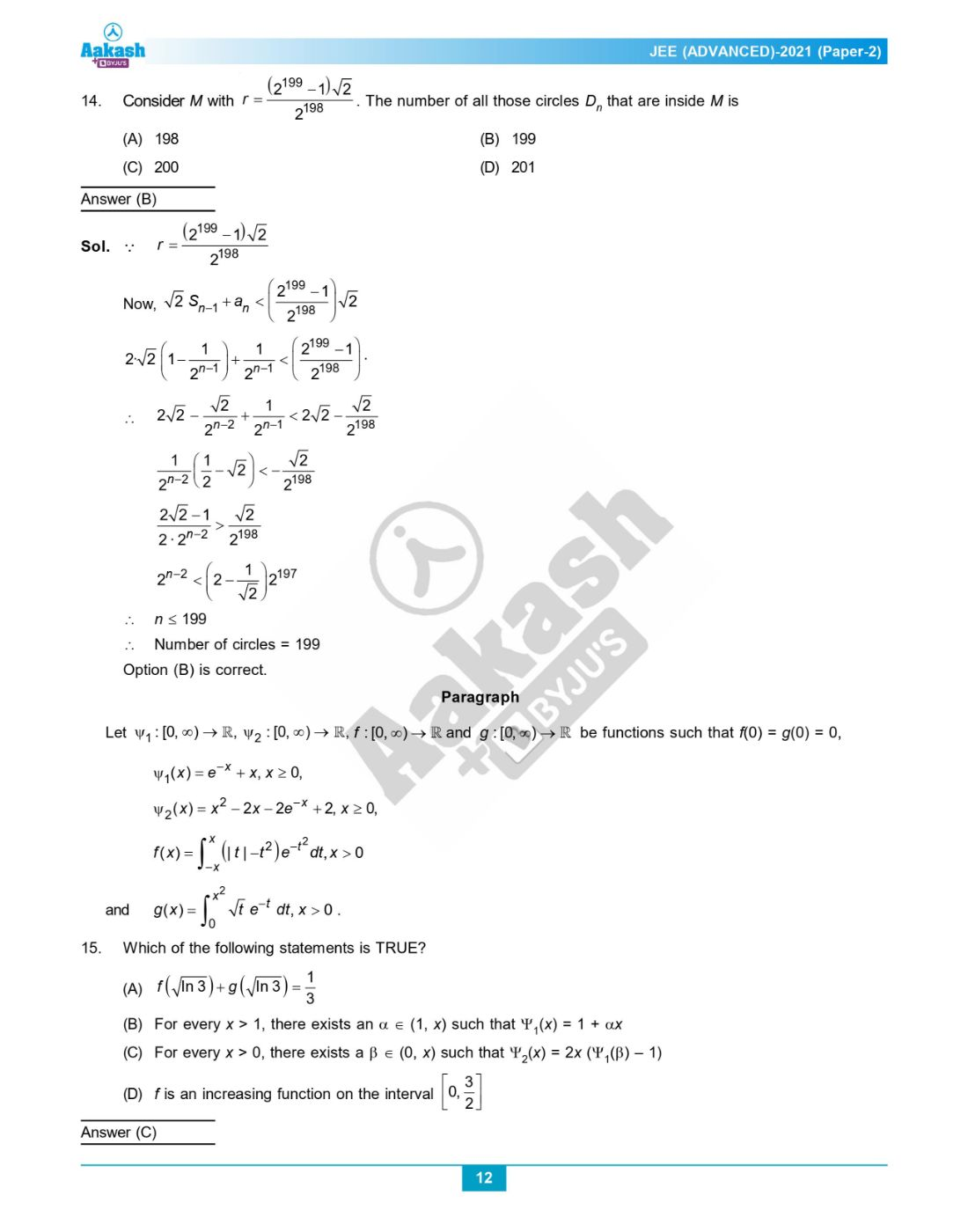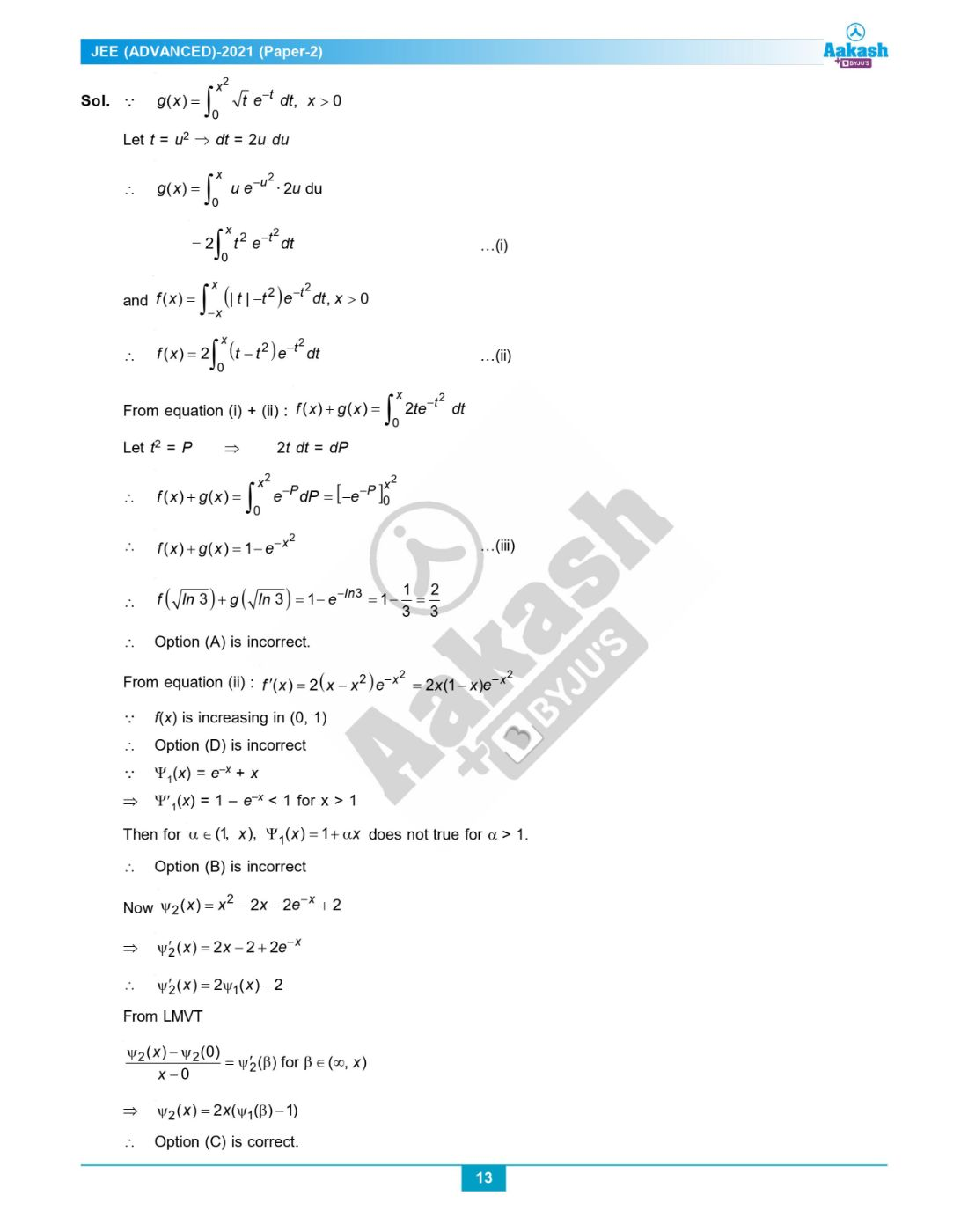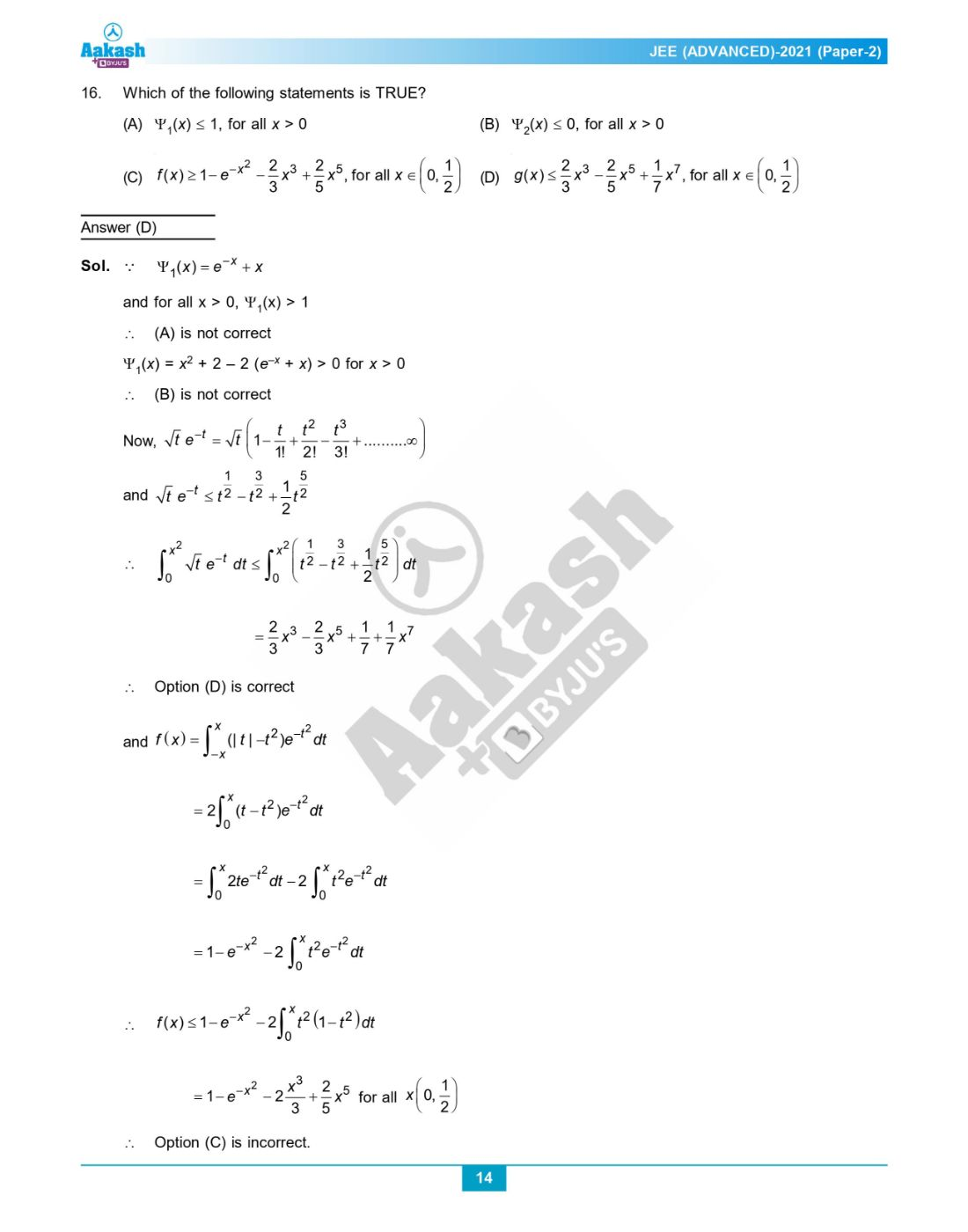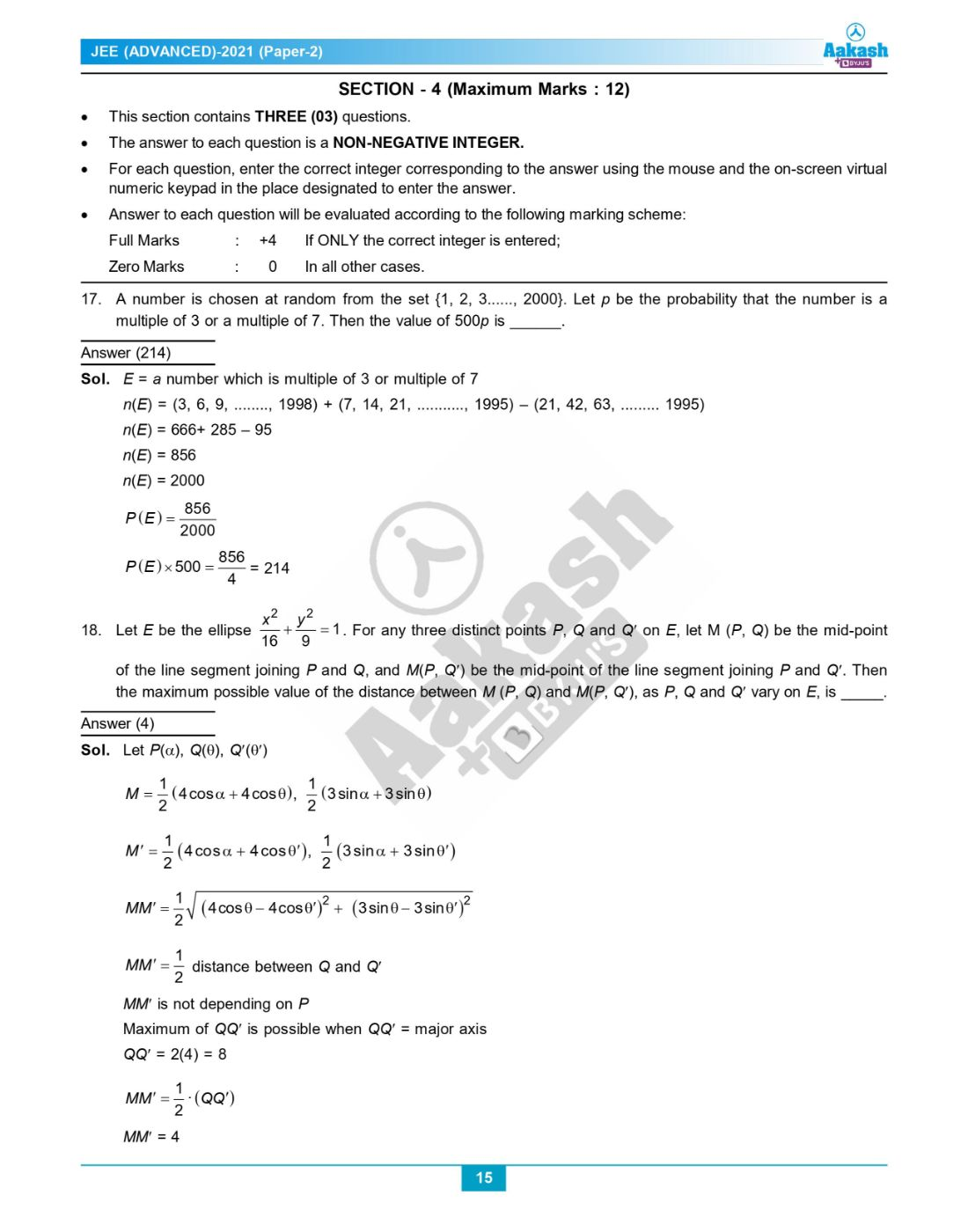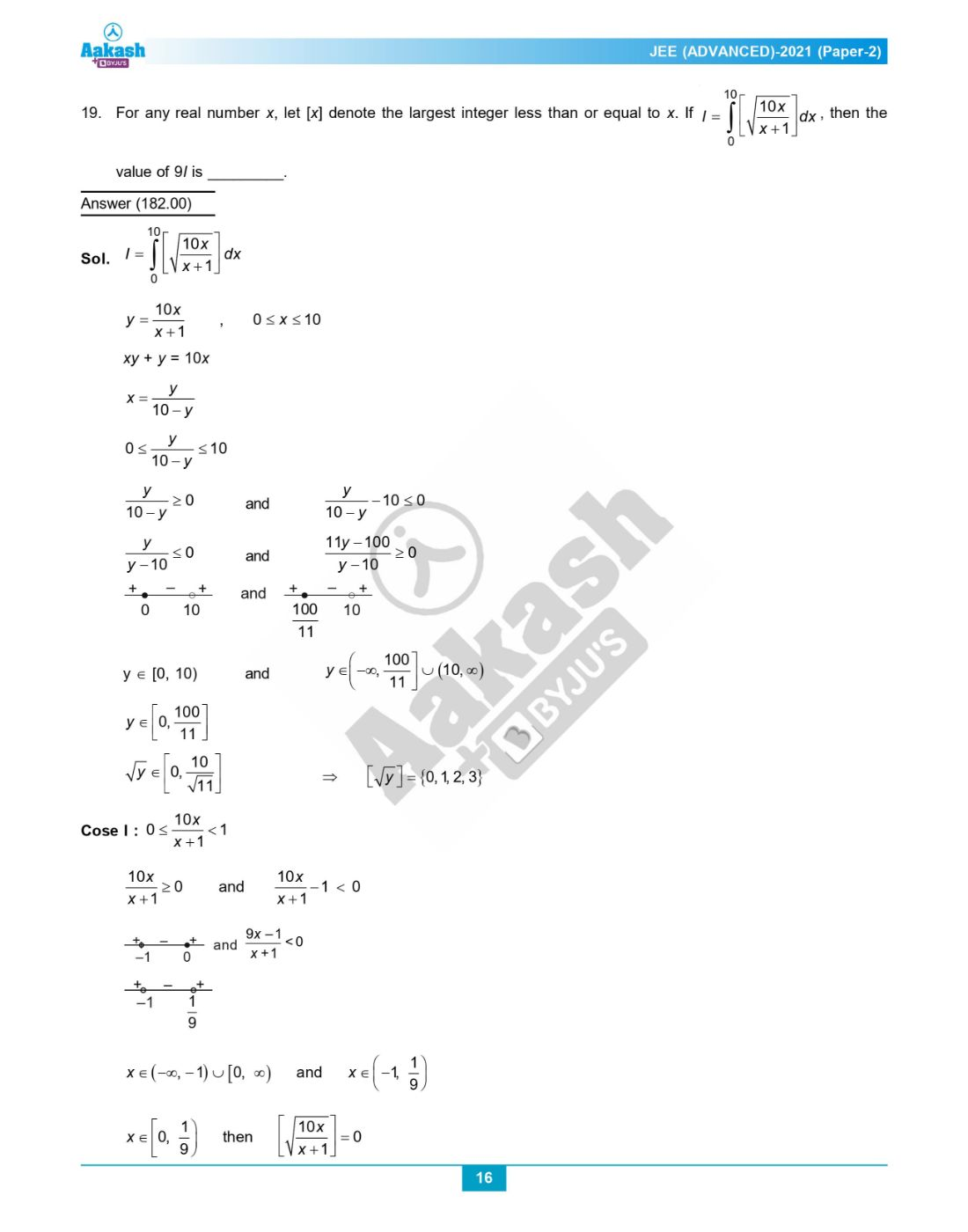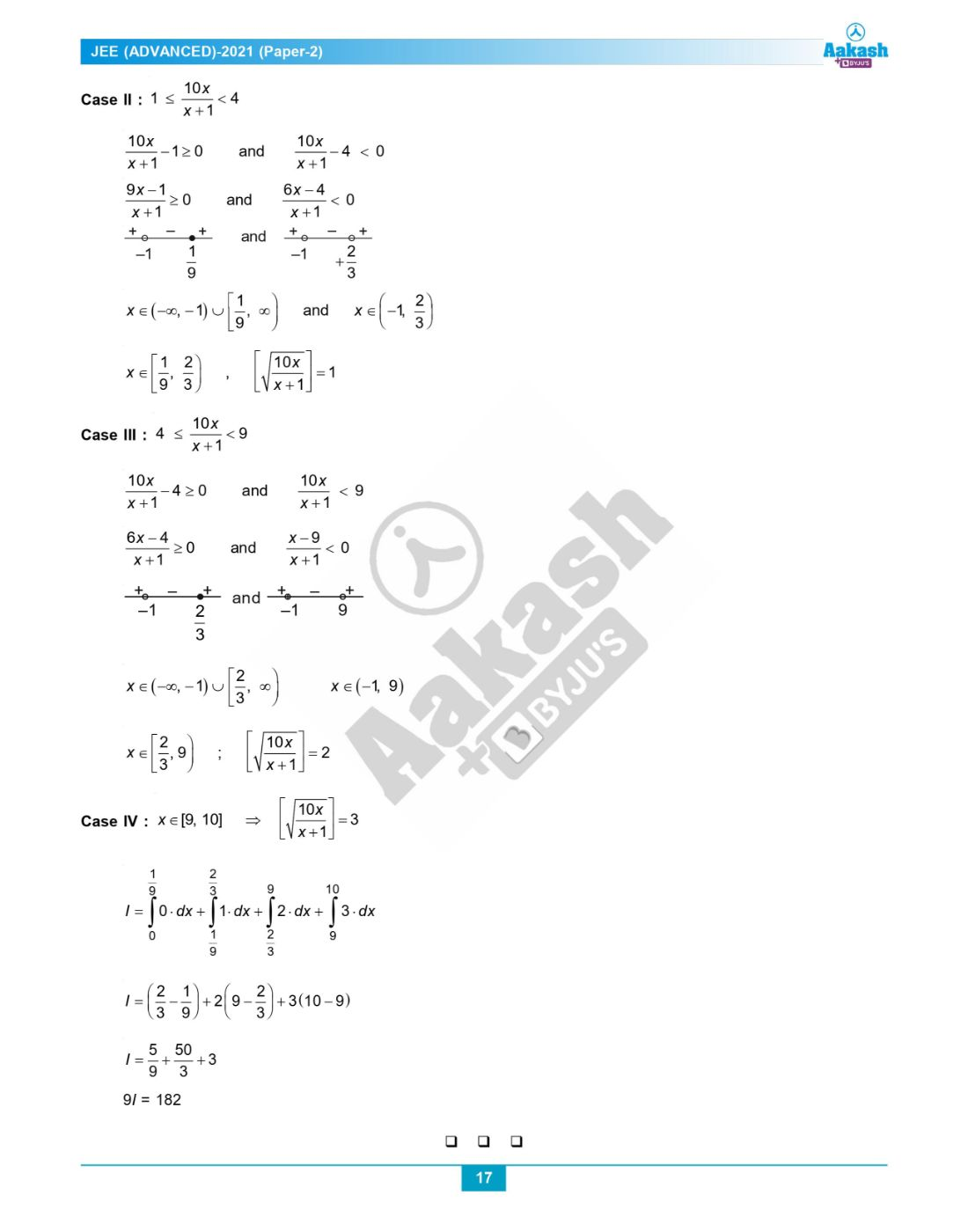Know more about the Repeaters Batch Know more about the Repeaters Batch

# JEE Advanced Question Paper 2021 Maths Paper 2

JEE Advanced 2021 Maths question paper for Paper 2 is available on this page. Students who are preparing for the entrance exam can refer to the questions and solutions given here to learn the answers in detail, get a better knowledge of the formulas used in a particular question and understand the proper logic behind solving each question. In essence, students will have a more effective exam preparation by solving the questions themselves. At the same time, they will be getting an idea of the important topics from an examination point of view.

Students can instantly download the solved JEE Advanced 2021 Maths question paper for Paper 2 in PDF format for offline practice. We have also provided analysis videos of both Paper 1 and Paper 2 for the exam that was held on October 3rd. Students will further get helpful insights about the paper pattern, question types, weightage of marks and more. More significantly, engaging in this study exercise will keep every JEE aspirant in good stead as the exam approaches nearer and nearer.

JEE Advanced 2021 Paper 2 Maths Question Paper

Question 1: Let;

S1 = {(i, j, k) : i, j, k ∈ {1,2,…,10}}

S2 = {(i, j) : 1 ≤ i < j + 2 ≤ 10,i, j ∈ {1,2,…,10}}

S3 = {(i, j, k, l) : 1 ≤ i < j < k < l, i, j, k, l ∈ {1,2,…,10}}

S4 = {(i, j,k,l ) : i, j, k and l are distinct elements in {1,2,…,10}}.

If the total number of elements in the set Sr is nr, r = 1, 2,3,4, then which of the following statements is (are) TRUE?

a. n1 = 1000
b. n2 = 44
c. n3 = 220
d. n4/12 = 420

Number of elements in S1 = 10 × 10 × 10 = 1000

Number of elements in S2 = 9 + 8 + 7 + 6 + 5 + 4 + 3 + 2 = 44

Number of elements in S3 = 10C4 = 210

Number of elements in S4 = 10P4 = 210 × 4! = 5040

Question 2: Consider a triangle PQR having sides of lengths p, q, and r opposite to the angles P, Q, and R, respectively. Then which of the following statements is (are) TRUE?

a. cos P ≥ 1 – p2/2qr
b. cos R ≥ ((q-r)/(p+q))cos P + ((p-r)/(p+q))cos Q
c. (q+r)/p < 2√(sin Q sin R)/sin P
d. if p<q and p<r, then cos Q > p/r and cos R > p/q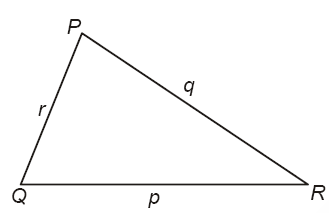(a) cos P = (q2+r2 – p2)/2qr

And (q2+r2)/2 ≥ √(q2. r2) (AM ≥ GM)

⇒ (q2+r2) ≥ 2qr

So cos P ≥ (2qr – p2)/2qr

cos P ≥ 1 – p2/2qr

(b) ((q-r) cos P + (p-r) cos Q)/(p+q) = ((q cos P + p cos Q) – r(cos P + cos Q))/(p+q)

= r(1- cos P – cos Q)/(p+q)

= (r(q-p cos R) – (p-q cos R))/(p+q)

= ((r-p-q)+(p+q)cos R)/(p+q)

= cos R + (r-q-p)/(p+q) ≤ cos R (since r < p+q)

(c) (q+r)/p = (sin Q + sin R)/sin P ≥ 2√(sin Q sin R)/sin P

(d) If p < q and q < r

So, p is the smallest side, therefore one of Q or R can be obtuse

So, one of cos Q or cos R can be negative

Therefore, cos Q > p/r and cos R > p/q cannot hold always.

Question 3: Let f: [-π/2, π/2] → R be a continuous function such that f(0) = 1 and ∫0π/3 f(t) dt = 0. Then which of the following statements is (are) TRUE?

a. The equation f(x) – 3 cos 3x = 0 has at least one solution in (0, π/3)
b. The equation f(x) – 3 sin 3x = -6/π has at least one solution in (0, π/3)
c.

$$\begin{array}{l}\lim_{x\to 0}\frac{x\int_{0}^{x}f(t)dt}{1-e^{x^{2}}}=-1\end{array}$$

d.
$$\begin{array}{l}\lim_{x\to 0}\frac{\sin x\int_{0}^{x}f(t)dt}{{x^{2}}}=-1\end{array}$$

f(0) = 1, ∫0π/3 f(t) dt = 0

(a) Consider a function g(x) = ∫0x f(t)dt – sin 3x. g(x) is continuous and differentiable function

And g(0) = 0

g(π/3) = 0

By Rolle’s theorem g’(x) = 0 has at least one solution in (0, π/3)

f(x) – 3 cos 3x = 0 for some x ∈ (0, π/3)

(b) Consider a function

h(x) = ∫0x f(t)dt + cos 3x + 6x/π

h(x) is continuous and differentiable function and h(0) = 1

h(π/3) = 1

By Rolle’s theorem h’(x) = 0 for at least one x ∈ (0, π/3)

f(x) – 3 sin 3x + 6/π = 0 for some x ∈ (0, π/3)

(c)

$$\begin{array}{l}\lim_{x\to 0}\frac{x\int_{0}^{x}f(t)dt}{1-e^{x^{2}}}\end{array}$$

(0/0 form)

By L’Hospital rule

=

$$\begin{array}{l}\lim_{x\to 0}\frac{xf(x)+\int_{0}^{x}f(t)dt}{-2xe^{x^{2}}}\end{array}$$
, (0/0 form)

=

$$\begin{array}{l}\lim_{x\to 0}\frac{xf'(x)+f(x)+f(x)}{-4x^{2}e^{x^{2}}-2e^{x^{2}}}\end{array}$$

= (0+2f(0))/(0-2)

= -1

(d)

$$\begin{array}{l}\lim_{x\to 0}\frac{\sin x\int_{0}^{x}f(t)dt}{{x^{2}}}\end{array}$$
, (0/0 form)

=

$$\begin{array}{l}\lim_{x\to 0}\frac{\sin x\: .f(x)+\cos x\int_{0}^{x}f(t)dt}{2x}\end{array}$$

=

$$\begin{array}{l}\lim_{x\to 0}\frac{\cos x\: .f(x)+\sin x.f'(x)+\cos x.f(x)-\sin x.\int_{0}^{x}f(t)dt}{2}\end{array}$$

= (1+0+1-0)/2

= 1

Question 4: For any real numbers α and β, let yα,β(x), x ∈ R, be the solution of the differential equation dy/dx + αy = xeβx, y(1) = 1. Let S = {yα,β(x), α, β ∈ R } . Then which of the following functions belong(s) to the set S?

a. f(x) = (x2/2)e-x + (e – ½)e-x
b. f(x) = (-x2/2)e-x + (e + ½)e-x
c. f(x) = (ex/2)(x-½) + (e – e2/4)e-x
d. f(x) = (ex/2)(½ -x) + (e + e2/4)e-x

dy/dx + αy = xeβx

Integrating factor (I.F) = e∫αdx = eαx

So, the solution is y.eαx = ∫xeβx eαx dx

y.eαx = ∫xe(β+α)x dx

If α + β ≠ 0

yeαx = x e(α+β)x/(α+β) – e(α+β)x/(α+β)2 + C

y = [eβx/(α+β)][x – 1/(α+β)] + Ce-αx …(i)

Put α = β = 1 in (i)

y = (ex/2)(x – ½) + Ce-x

y(1) = 1

1 = (e/2)(½) +C/e

⇒ C = e – e2/4

So, y = (ex/2)(x-½) + (e – e2/4)e-x

If α + β = 0 and α = 1

dy/dx + y = xe-x

I.F = ex

yex = ∫x dx

yex = x2/2 + C

y = e-xx2/2 + Ce-x

y(1) = 1

1 = 1/2e + C/e

⇒ C = e – ½

y = e-xx2/2 + (e – ½)e-x

Question 5: Let O be the origin and

$$\begin{array}{l}\overrightarrow{OA}=2\hat{i}+2\hat{j}+\hat{k}\end{array}$$
,
$$\begin{array}{l}\overrightarrow{OB}=\hat{i}-2\hat{j}+2\hat{k}\end{array}$$
and
$$\begin{array}{l}\overrightarrow{OC}=\frac{1}{2}(\overrightarrow{OB}-\lambda \overrightarrow{OA})\end{array}$$
for some λ> 0. If
$$\begin{array}{l}\left | \overrightarrow{OB}\times \overrightarrow{OC}\right |=\frac{9}{2}\end{array}$$
, then which of the following statements is (are) TRUE ?

a. Projection of

$$\begin{array}{l}\overrightarrow{OC}\end{array}$$
on
$$\begin{array}{l}\overrightarrow{OA}\end{array}$$
is -3/2

b. Area of the triangle OAB is 9/2
c. Area of the triangle ABC is 9/2
d. The acute angle between the diagonals of the parallelogram with adjacent sides
$$\begin{array}{l}\overrightarrow{OA}\end{array}$$
and
$$\begin{array}{l}\overrightarrow{OC}\end{array}$$
is π/3

$$\begin{array}{l}\overrightarrow{OA}=2\hat{i}+2\hat{j}+\hat{k}\end{array}$$
$$\begin{array}{l}\overrightarrow{OB}=\hat{i}-2\hat{j}+2\hat{k}\end{array}$$
$$\begin{array}{l}\overrightarrow{OC}=\frac{1}{2}(\overrightarrow{OB}-\lambda \overrightarrow{OA})\end{array}$$
$$\begin{array}{l}\overrightarrow{OB}\times \overrightarrow{OC}=\overrightarrow{OB}\times \frac{1}{2}(\overrightarrow{OB}-\lambda \overrightarrow{OA})\end{array}$$

=

$$\begin{array}{l}\frac{-\lambda }{2}\overrightarrow{OB}\times \overrightarrow{OA}=\frac{\lambda }{2}(\overrightarrow{OA}\times \overrightarrow{OB})\end{array}$$

Now,

$$\begin{array}{l}\overrightarrow{OA}\times \overrightarrow{OB}=\begin{vmatrix} \hat{i} & \hat{j} & \hat{k}\\ 2& 2 &1 \\ 1& -2&2 \end{vmatrix}=6\hat{i}-3\hat{j}-6\hat{k}\end{array}$$

So,

$$\begin{array}{l}\overrightarrow{OB}\times \overrightarrow{OC}=\frac{3\lambda }{2}(2\hat{i}-\hat{j}-2\hat{k})\\\end{array}$$
$$\begin{array}{l}\left | \overrightarrow{OB}\times \overrightarrow{OC} \right |=\left | \frac{9\lambda }{2} \right |=\frac{9}{2}\end{array}$$

So, λ = 1 (since λ >0)

$$\begin{array}{l}\overrightarrow{OC}=\frac{1}{2}(\overrightarrow{OB}-\overrightarrow{OA})\\\end{array}$$
$$\begin{array}{l}\overrightarrow{OC}=\frac{1}{2}(-\hat{i}-4\hat{j}+\hat{k})\\\end{array}$$

(a) Projection of vector OC on vector OA =

$$\begin{array}{l}\frac{\overrightarrow{OC}.\overrightarrow{OA}}{\left | \overrightarrow{OA} \right |}\end{array}$$

= ½ (-2-8+1)/3

= -3/2

(b) Area of triangle OAB =

$$\begin{array}{l}\frac{1}{2}\left | \overrightarrow{OA}\times \overrightarrow{OB} \right |\end{array}$$
= 9/2

(c) Area of the triangle ABC is =

$$\begin{array}{l}\frac{1}{2}\left | \overrightarrow{AB}\times \overrightarrow{AC} \right |=\frac{1}{2}\left |\begin{vmatrix} \hat{i} & \hat{j}&\hat{k} \\ -1& -4 & 1\\ -\frac{5}{2}& -4 &- \frac{1}{2} \end{vmatrix} \right |\end{array}$$

=

$$\begin{array}{l}\frac{1}{2}\left | 6\hat{i}-3 \hat{j}-6\hat{k}\right |\end{array}$$

= 9/2

(d) Acute angle between the diagonals of the parallelogram with adjacent sides

$$\begin{array}{l}\overrightarrow{OA}\end{array}$$
and
$$\begin{array}{l}\overrightarrow{OC}\end{array}$$
= θ

$$\begin{array}{l}\frac{(\overrightarrow{OA}+\overrightarrow{OC}).(\overrightarrow{OA}-\overrightarrow{OC})}{\left | \overrightarrow{OA}+\overrightarrow{OC}\right |\left | \overrightarrow{OA}-\overrightarrow{OC}\right |}=\cos \theta\end{array}$$

cos θ =

$$\begin{array}{l}\frac{(\frac{3}{2}\hat{i}+\frac{3}{2}\hat{k}).(\frac{5}{2}\hat{i}+4\hat{j}+\frac{1}{2}\hat{k})}{\frac{3}{2}\sqrt{2}\times \sqrt{\frac{90}{4}}}\end{array}$$

= 18/3√2 ×√90

θ ≠ π/3

Question 6: Let E denote the parabola y2 = 8x. Let P = (-2, 4), and let Q and Q’ be two distinct points on E such that the lines PQ and PQ’ are tangents to E. Let F be the focus of E. Then which of the following statements is (are) TRUE?

a. The triangle PFQ is a right-angled triangle
b. The triangle QPQ’ is a right-angled triangle
c. The distance between P and F is 5√2
d. F lies on the line joining Q and Q’

E : y2 = 8x

P : (-2, 4)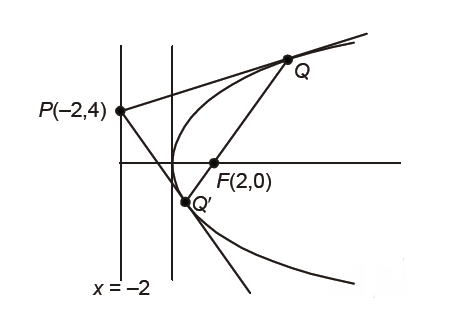Point P (-2, 4) lies on directrix (x = -2) of parabola y2 = 8x

So, ∠QPQ’ = π/2 and chord QQ’ is a focal chord and segment PQ subtends a right angle at the focus.

Slope of QQ’ = 2/(t1+t2) = 1

Slope of PF = -1

PF = 4√2

Question Stem for Question Nos. 7 and 8

Consider the region R = {(x,y)∈ R×R : x ≥ 0 and y2 ≤ 4 – x. Let F be the family of all circles that are contained in R and have centres on the x-axis. Let C be the circle that has the largest radius among the circles in F. Let (α, β) be a point where circle C meets the curve y2 = 4 – x.

Question 7: The radius of the circle C is

Question 8: The value of α is

Sol: For comprehension Question 7 and Question 8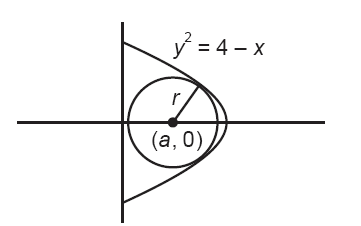Let the circle be,

(x – a)2 + y2 = r2

Solving it with parabola

y2 = 4 – x we get

(x – a)2 + 4 – x = r2

x2 – x(2a + 1) + (a2 + 4 – r2) = 0 …(1)

D = 0

⇒ 4r2 + 4a – 15 = 0

Clearly a ≥ r

So 4r2 + 4r – 15 ≤ 0

⇒ rmax = 3/2 = a

Radius of circle C is 3/2

From (1) x2 – 4x + 4 = 0

⇒ x = 2 = α

Question Stem for Question Nos. 9 and 10

Let f1 : (0, ∞) → R and f2 : (0, ∞) → R be defined by f1(x) =

$$\begin{array}{l}\int_{0}^{x}\prod_{j=1}^{21}(t-j)^{j}dt\end{array}$$
, x>0 and f2(x) = 98(x – 1)50 – 600(x – 1)49 + 2450, x > 0, where, for any positive integer n and real number a1, a2,…an.
$$\begin{array}{l}\prod_{i=1}^{n}a_{i}\end{array}$$
denotes the product of a1, a2,..an. Let mi and ni, respectively, denote the number of points of local minima and the number of points of local maxima of function fi, i = 1, 2, in the interval (0, ∞).

Question 9: The value of 2m1 + 3n1 + m1n1 is

Question 10: The value of 6m2 + 4n2 + 8m2n2 is

Solution for Question 9 and 10

$$\begin{array}{l}f_{1}'(x)=\prod_{j=1}^{21}(x-j)^{j}\\\end{array}$$

f1’(x) = (x – 1)(x – 2)2 (x – 3)3 ,…, (x – 20)20 (x – 21)21

Checking the sign scheme of f1’(x) at x = 1, 2, 3, …, 21, we get

f1(x) has local minima at x = 1, 5, 9, 13, 17, 21 and local maxima at x = 3, 7, 11, 15, 19

⇒ m1 = 6, n1 = 5

f2(x) = 98(x – 1)50 – 600(x – 1)49 + 2450

f2’(x) = 98 × 50(x – 1)49– 600 × 49 × (x – 1)48

= 98 × 50 × (x – 1)48 (x – 7)

f2(x) has local minimum at x = 7 and no local maximum.

⇒ m2 = 1, n2 = 0

2m1 + 3n1 + m1n1

= 2 × 6 + 3 × 5 + 6 × 5

= 57

6m2 + 4n2 + 8m2n2

= 6 × 1 + 4 × 0 + 8 × 1 × 0

= 6

Question Stem for Question Nos. 11 and 12

Let gi = [π/8, 3π/8] → R, i = 1, 2 and f: [π/8, 3π/8] → R be the functions such that g1(x) = 1, g2(x) = |4x – π| and f(x) = sin2x, for all x∈[π/8, 3π/8]. Define

$$\begin{array}{l}S_{i}=\int_{\frac{\pi }{8}}^{\frac{3\pi }{8}}f(x).g_{i}(x)dx\end{array}$$
, i = 1,2.

Question 11: The value of 16S1/π is

S1 = ∫π/83π/8 sin2x . 1 dx

= ½ ∫π/83π/8(1 – cos 2x)dx

= ½ (x – sin 2x/x)π/83π/8

= ½ (π/4 – 0)

= π/8

=> 16S1/π = 2

Question 12:The value of 48S22 is

S2= ∫π/83π/8 sin2x |4x – π| dx

= ∫π/83π/8 4 sin2x |x – π/4| dx

Let x – π/4 = t

=> dx = dt

S2 = ∫-π/8π/8 4 sin2 (π/4 + t)|t| dt

= ∫-π/8π/8 2(1 – cos 2(π/4 + t) |t| dt

= ∫-π/8π/8 (2 + 2 sin 2t) |t| dt

= 2∫-π/8π/8|t|dt + 2∫-π/8π/8|t| sin 2t dt

= 4∫0π/8t dt + 0

S2 = [2t2]0π/8

= π2/32

48S22 = 3/2

Question Paragraph: Let M = {(x, y) ∈ R × R : x2 + y2≤ r2}, where r > 0. Consider the geometric progression an = 1/2n-1, n = 1,2, 3… Let S0 = 0 and, for n ≥ 1, let Sn denote the sum of the first n terms of this progression. For n ≥ 1 , let Cn denote the circle with center (Sn–1, 0) and radius an, and Dn denote the circle with center (Sn–1, Sn–1) and radius an.

Question 13: Consider M with r = 1025/513. Let k be the number of all those circles Cn that are inside M. Let l be the maximum possible number of circles among these k circles such that no two circles intersect. Then,

a. k + 2l = 22
b. 2k + l = 26
c. 2k + 3l = 34
d. 3k + 2l = 40

an = 1/2n-1

And Sn = 2(1 – 1/2n)

For circles Cn to be inside M.

Sn-1 + an < 1025/513

⇒ Sn < 1025/513

⇒ 1 – 1/2n < 1025/1026

⇒ 1 – 1/1026

⇒ 2n < 1026

⇒ n ≤ 10

∴ Number of circles inside be 10 = K

Clearly, alternate circles do not intersect each other i.e., C1, C3, C5, C7, C9 do not intersect each other as well as C2, C4, C6, C8 and C10 do not intersect each other hence maximum of 5 set of circles do not intersect each other.

∴ l = 5

∴ 3K + 2l = 40

∴ Option (D) is correct

Question 14: Consider M with r = (2199-1)√2/2198. The number of all those circles Dn that are inside M is;

a. 198
b. 199
c. 200
d. 201

Since r = (2199-1)√2/2198

Now, √2Sn-1 + an < (2199-1)√2/2198

2√2(1 – 1/2n-1) + 1/2n-1 < (2199-1)/2198

∴ 2√2 – √2/2n-2 + 1/2n-1 < 2√2 – √2/2198

(1/2n-2)(½ – √2) < -√2/2198

(2√2-1)/2. 2n-2 > √2/2198

2n-2 < (2 – 1/√2) 2197

n ≤ 199

∴ Number of circles = 199

Option (B) is correct.

Question Paragraph: Let ψ1 = [0, ∞) → R, ψ2 = [0, ∞) → R, f:[0, ∞) → R and g:[0, ∞) → R be functions such that f(0) = g(0) = 0,

ψ1(x) = e-x + x, x≥0,

ψ2(x) = x2 – 2x – 2e-x + 2, x≥0

$$\begin{array}{l}f(x)=\int_{-x}^{x}(\left | t \right |-t^{2})e^{-t^{2}}dt, x> 0\end{array}$$

And

$$\begin{array}{l}g(x)=\int_{0}^{x^{2}}\sqrt{t}e^{-t}dt,x> 0\end{array}$$

Question 15: Which of the following statements is TRUE?

a. f(√ln 3) + g(√ln 3) = ⅓
b. For every x > 1, there exists an α ∈ (1, x) such that ψ1(x) = 1 + α x
c. For every x > 0, there exists a β ∈ (0, x) such that ψ2(x) = 2x (ψ1(β) -1)
d. f is an increasing function on the interval [0, 3/2]

Since,

$$\begin{array}{l}g(x)=\int_{0}^{x^{2}}\sqrt{t}e^{-t}dt,x> 0\end{array}$$

Let t = u2

⇒ dt = 2u du

So g(x) =

$$\begin{array}{l}\int_{0}^{x}ue^{-u^{2}}.2u\: du\\\end{array}$$

=

$$\begin{array}{l}2\int_{0}^{x}t^{2}e^{-t^{2}}dt\\\end{array}$$
…(i)

And

$$\begin{array}{l}f(x)=\int_{-x}^{x}(\left | t \right |-t^{2})e^{-t^{2}}dt, x> 0\end{array}$$

Therefore

$$\begin{array}{l}f(x)=2\int_{0}^{x}(t-t^{2})e^{-t^{2}}dt\end{array}$$
…(ii)

From equation (i) + (ii) : f(x) + g(x) =

$$\begin{array}{l}\int_{0}^{x}2te^{-t^{2}}dt\end{array}$$

Let t2 = P

⇒ 2t dt = dP

f(x) + g(x) =

$$\begin{array}{l}\int_{0}^{x^{2}}e^{-P}dP= \left [ -e^{-P} \right ]_{0}^{x^{2}}\end{array}$$

f(x) + g(x) =

$$\begin{array}{l}1-e^{-x^{2}}\end{array}$$
…(iii)

∴ f(√ln 3) + g(√ln 3) = 1-e-ln 3

= 1-⅓

= ⅔

∴ Option (a) is incorrect.

From equation (ii) : f’(x) =

$$\begin{array}{l}f'(x)=2(x-x^{2})e^{-x^{2}}=2x(1-x)e^{-x^{2}}\end{array}$$

Since f(x) is increasing in (0, 1)

∴ Option (d) is incorrect

ψ1(x) = e-x + x

⇒ ψ1’(x) = 1- e-x <1 for x>1

Then for α ∈ (1, x), ψ1(x) = 1+ αx does not true for α > 1.

∴ Option (b) is incorrect

Now ψ2(x) = x2 – 2x – 2e-x + 2

ψ2’(x) = 2x – 2 + 2e-x

∴ ψ2’(x) = 2ψ1(x)-2

From LMVT

2(x) – ψ2(0)]/(x-0) = ψ2’(β) for β ∈(∞, x)

=> ψ2(x) = 2x(ψ1(β) – 1)

Option (c) is correct.

Question 16: Which of the following statements is TRUE?

a. ψ1(x) ≤ 1, for all x>0
b. ψ2(x) ≤ 0, for all x>0
c.

$$\begin{array}{l}f(x)\geq 1-e^{-x^{2}}-\frac{2}{3}x^{3}+\frac{2}{5}x^{5}\end{array}$$
, for all x∈(0, ½)

d. g(x)≤ (2/3) x3 – (⅖)x5 + (1/7)x7, for all x∈(0, ½)

Since ψ1(x) = e-x+x

And for all x>0, ψ1(x) > 1

∴ (a) is not correct

ψ2(x) = x2 + 2 – 2(e-x + x) >0 for x > 0

∴ (b) is not correct.

Now, √te-t = √t(1 – t/1! + t2/2! – t3/3! + ….∞)

And √te-t ≤ t1/2 – t3/2 + ½ t5/2

$$\begin{array}{l}\int_{0}^{x^{2}}\sqrt{t}e^{-t}dt\leq \int_{0}^{x^{2}}(t^{\frac{1}{2}}-t^{\frac{3}{2}}+\frac{1}{2}t^{\frac{5}{2}})dt\end{array}$$

= (⅔) x3 – (⅔) x5 + (1/7) + (1/7) x7

∴ Option (d) is correct

And

$$\begin{array}{l}f(x)=\int_{-x}^{x}(\left | t \right |-t^{2})e^{-t^{2}}dt\end{array}$$

=

$$\begin{array}{l}2\int_{0}^{x}(t-t^{2})e^{-t^{2}}dt\end{array}$$

=

$$\begin{array}{l}2\int_{0}^{x}2te^{-t^{2}}dt-2\int_{0}^{x}t^{2}e^{-t^{2}}dt\\\end{array}$$

=

$$\begin{array}{l}1-e^{-x^{2}}-2\int_{0}^{x}t^{2}e^{-t^{2}}dt\end{array}$$

Therefore

$$\begin{array}{l}f(x)\leq 1-e^{-x^{2}}-2\int_{0}^{x}t^{2}(1-t^{2})dt\end{array}$$

=

$$\begin{array}{l}1-e^{-x^{2}}-2\frac{x^{3}}{3}+\frac{2}{5}x^{5}\end{array}$$
for all x∈(0, ½)

∴ Option (c) is incorrect.

Question 17: A number is chosen at random from the set {1, 2, 3……, 2000}. Let p be the probability that the number is a multiple of 3 or a multiple of 7. Then the value of 500p is;

E = a number which is multiple of 3 or multiple of 7

n(E) = (3, 6, 9, …….., 1998) + (7, 14, 21, ……….., 1995) – (21, 42, 63, ……… 1995)

n(E) = 666+ 285 – 95

n(E) = 856

n(E) = 2000

P(E) = 856/2000

P(E) × 500 = 856/4 = 214

Question 18: Let E be the ellipse x2/16 + y2/9 = 1. For any three distinct points P, Q and Q’ on E, let M (P, Q) be the mid-point of the line segment joining P and Q, and M(P, Q’) be the mid-point of the line segment joining P and Q’. Then the maximum possible value of the distance between M (P, Q) and M(P, Q’), as P, Q and Q’ vary on E, is

Let P(α), Q(θ), Q’(θ’)

M = ½ (4 cos α + 4 cos θ), ½ (3 sin α + 3 sin θ)

M’ = ½ (4 cos α + 4 cos θ’), ½ (3 sin α + 3 sin θ’)

MM’ = ½ √((4 cos θ – 4 cos θ’)2 + (3 sin θ – 3 sin θ’)2)

MM’ = ½ distance between Q and Q’

MM’ is not depending on P

Maximum of QQ’ is possible when QQ’ = major axis

QQ’ = 2(4) = 8

MM’ = ½ (QQ’)

MM’ = 4

Question 19: For any real number x, let [x] denote the largest integer less than or equal to x. If

$$\begin{array}{l}l=\int_{0}^{10}\left [ \sqrt{\frac{10x}{x+1}} \right ]dx\end{array}$$
, then the value of 9I is;

$$\begin{array}{l}l=\int_{0}^{10}\left [ \sqrt{\frac{10x}{x+1}} \right ]dx\end{array}$$

y = 10x/(x+1), 0 ≤ x≤10

xy+y = 10x

x = y/(10-y)

0≤ y/(10-y) ≤ 10

y/(10-y) ≥ 0 and (y/(10-y)) – 10 ≤ 0

y/(y-10) ≤ 0 and (11y-100)/(y-10) ≥ 0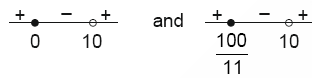y ∈ [0, 10) and y ∈ (-∞, 100/11] ⋃ (10, ∞)

y ∈ [0, 100/11]

√y ∈ [0, 10/√11]

⇒ [√y] = {0, 1, 2, 3}

Case 1:

0≤10x/(x+1) < 1

10x/(x+1) ≥ 0 and 10x/(x+1) – 1 < 0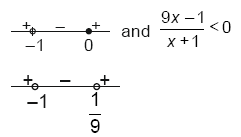x∈ (-∞, -1) ⋃ [0, ∞) and x∈ (-1, 1/9)

x∈ [0, 1/9) then [√(10x/(x+1))] = 0

Case 2:

1 ≤ 10x/(x+1) < 4

10x/(x+1) – 1 ≥ 0 and 10x/(x+1) – 4 < 0

(9x-1)/(x+1) ≥ 0 and (6x-4)/(x+1) < 0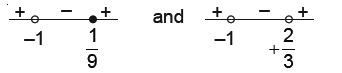x∈ (-∞, -1)⋃ [1/9, ∞) and x∈ (-1, ⅔)

x∈[1/9, ⅔), [√(10x/(x+1))] = 1

Case 3:

4 ≤ (10x/x+1) < 9

10x/(x+1) – 4 ≥ 0 and 10x/(x+1) < 9

(6x-4)/(x+1) ≥ 0 and (x-9)/(x+1) < 0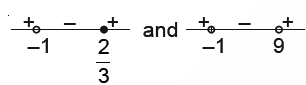x∈ (-∞, -1)⋃ [2/3, ∞) and x∈ (-1, 9)

x∈ [⅔, 9); [√(10x/(x+1))] = 2

Case 4:

x∈ [9, 10]

⇒ [√(10x/(x+1))] = 3

l =

$$\begin{array}{l}\int_{0}^{\frac{1}{9}}0.dx +\int_{\frac{1}{9}}^{\frac{2}{3}}1.dx+\int_{\frac{2}{3}}^{9}2.dx+\int_{9}^{10}3.dx\end{array}$$

l = (⅔ – 1/9) +2(9-⅔) + 3(10-9)

l = 5/9 + 50/3 + 3

9l = 182